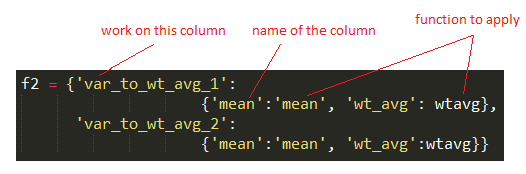# weighted avarage, aggrefated function with apply and agg

import numpy as np
import pandas as pd
from IPython.display import display


### 1. weighted average

Let's denote x = [x_1, ..., x_n]. The weight w is denoted as w = [w_1, ..., w_n]. The weighted average of x by w is $\frac{ \sum_{i=1}^{n} x_i * w_i } { \sum_{i=1}^{n} w_i}$

numpy provides a function called np.average() to calculate the weighted average. An example of calculate by hand and by the np.average is given below:

np.random.seed(9999)
df = pd.DataFrame(np.random.random(20).reshape(10, 2), columns = ['val1', 'wt'])

# if we want to calcuate the weighted average of val1 weighted by wt, that is:
sum(df.val1 * df.wt) / sum(df.wt)    # 0.42105022244260254

# np.average() function
np.average(df.val1, weights = df.wt, axis = 0)    # 0.42105022244260254

0.42105022244260254


### 2. calculate weighted average within each group

The above example is very simple. A more common situation is there are different groups, and we need to calculate the weighted average within each group. The following is an example:

1. there are two groups, called 'id'
2. we want to calculate the weighted average for data in group 1(id == 1) and group 2(id == 2)
3. calculate the weighted average of var1 and var2 by wt in group 1, and group 2 seperately

so, 0.339688030253 = sum(df1.val1 * df1.wt) / df1.wt.sum()

np.random.seed(9999)
df = pd.DataFrame(np.random.random(20).reshape(10, 2), columns = ['val1', 'val2'])
df['id'] = np.repeat([1, 2], 5)
df['wt'] = [1, 2] * 5

print df

print '\n' + '-'*20 + ' weighted average of val1 and val2 by wt in each group ' + '-'*20

wtavg = lambda x: np.average(x.ix[:, :2], weights = x.wt, axis = 0)
dfwavg = df.groupby('id').apply(wtavg)
display(dfwavg)

       val1      val2  id  wt
0  0.823389  0.218603   1   1
1  0.031461  0.545926   1   2
2  0.154456  0.186963   1   1
3  0.422348  0.605552   1   2
4  0.492354  0.644119   1   1
5  0.913049  0.771104   2   2
6  0.288361  0.415441   2   1
7  0.331490  0.366327   2   2
8  0.312195  0.580446   2   1
9  0.112952  0.325875   2   2

-------------------- weighted average of val1 and val2 by wt in each group --------------------

id
1    [0.339688030253, 0.478948919665]
2     [0.41444248036, 0.490312314239]
dtype: object

## verified by manual calculation
df1 = df.query('id == 1')
sum(df1.val1 * df1.wt) / df1.wt.sum()    ## 0.33968803025301908

0.33968803025301908


Difference between apply and agg: apply will apply the funciton on the data frame of each group, while agg will aggregate each column of each group. So the arguments in the apply function is a dataframe.

The following is an example from pandas docs. The arguments in function f0 is a dataframe in each id group.

def f0(group):
return pd.DataFrame({'original': group, 'group - mean': group - group.mean()})

df.groupby('id')['val1'].apply(f0)

group - mean original
0 0.438588 0.823389
1 -0.353340 0.031461
2 -0.230346 0.154456
3 0.037546 0.422348
4 0.107553 0.492354
5 0.521440 0.913049
6 -0.103248 0.288361
7 -0.060119 0.331490
8 -0.079414 0.312195
9 -0.278658 0.112952

### multiple functions

#### 1. different function for different column

One condition is you want to apply different function on different columns in the dataframe. For example, you want to apply sum on one column, and stdev on another column.

if you want to apply multiple functions to aggregate, then you need to put them in the list or dict.

np.random.seed(9999)
snapdf = pd.DataFrame(np.random.random(3000).reshape(-1, 3), \
columns = ['wt_var', 'var_to_sum', "var_to_stdev"])
snapdf['stage'] = np.repeat([1, 2], 500)

f = {"var_to_sum": np.sum, "var_to_stdev": np.std}
stage_s1 = snapdf.ix[:, ['stage', 'var_to_sum', 'var_to_stdev']].groupby('stage').agg(f).reset_index()

stage_s1

stage var_to_sum var_to_stdev
0 1 245.666111 0.288775
1 2 247.715539 0.284739

#### 2. multiple functions on one column

Another condition is you want to apply multiple functions on each column. For example, you want to calcualte both mean and stdev for eachc column. Then your function is a dict of dicts: first key is the column(variable) to be applied by the functions. second key is the functions.

An example is like:

np.random.seed(9999)
snapdf = pd.DataFrame(np.random.random(3000).reshape(-1, 3), \
columns = ['wt_var', 'var1', "var2"])
snapdf['stage'] = np.repeat([1, 2], 500)

snapdf.groupby('stage').agg({np.sum, np.mean, np.std})

wt_var var1 var2
std mean sum std mean sum std mean sum
stage
1 0.27400 0.484682 242.341116 0.287491 0.491332 245.666111 0.288775 0.506056 253.028070
2 0.29702 0.507317 253.658370 0.282293 0.495431 247.715539 0.284739 0.501093 250.546638
np.random.seed(9999)
snapdf = pd.DataFrame(np.random.random(3000).reshape(-1, 3), \
columns = ['wt_var', 'var_to_wt_avg_1', 'var_to_wt_avg_2'])
snapdf['stage'] = np.repeat([1, 2], 500)

wtavg = lambda x: np.average(x, weights = snapdf.loc[x.index, "wt_var"])

f2 = {'var_to_wt_avg_1': {'mean':'mean', 'wt_avg': wtavg}, 'var_to_wt_avg_2':{'mean':'mean', 'wt_avg':wtavg}}
stage_s2 = snapdf.ix[:, ['stage', 'var_to_wt_avg_1', 'var_to_wt_avg_2']].groupby('stage').agg(f2).reset_index()

# stage_s2.columns = stage_s2.columns.droplevel(0)

stage_s2stage var_to_wt_avg_2 var_to_wt_avg_1
wt_avg mean wt_avg mean
0 1 0.498908 0.506056 0.486139 0.491332
1 2 0.517657 0.501093 0.499796 0.495431

### 3. weighted from stacked data: unstack data

Data in the previous are stored in horizontial way: variables are in different columns. But sometimes data are in vertical way: in the following example, the 3 ratings(I/S/P) are stacked in vertical line. We want to get the weighted average of I/S/P. So, the first step is to unstak the data. Then calculate the weighted average.

np.random.seed(9999)
df = pd.DataFrame(np.random.random(72).reshape(36, 2), columns = ["var1", "var2"])
df["rating"] = np.repeat(['I', 'S', 'P'], 12)
df['qtrs'] = range(1, 13) * 3

df = df.set_index(['rating', 'qtrs']).unstack(level = 0)
df['cnts', 'I'] = 100
df['cnts', 'P'] = 200
df['cnts', "S"] = 300

df['wt_var_1'] = np.average(df.var1, weights = df.cnts, axis = 1)
df['wt_var_2'] = np.average(df.var2, weights = df.cnts, axis = 1)

df.reset_index().ix[:, ["qtrs", "wt_var_1", "wt_var_2"]]

var1 var2 cnts
rating I P S I P S I P S
qtrs
1 0.823389 0.154764 0.602518 0.218603 0.904529 0.435665 100 200 300
2 0.031461 0.365481 0.472321 0.545926 0.580417 0.866001 100 200 300
3 0.154456 0.494557 0.537686 0.186963 0.824609 0.484590 100 200 300
4 0.422348 0.503091 0.333305 0.605552 0.670854 0.582543 100 200 300
5 0.492354 0.446044 0.679368 0.644119 0.050341 0.544653 100 200 300
qtrs wt_var_1 wt_var_2
rating
0 1 0.490078 0.555776
1 2 0.363231 0.717461
2 3 0.459438 0.548325
3 4 0.404741 0.615815
4 5 0.570425 0.396460
5 6 0.870236 0.845366
6 7 0.446611 0.123709
7 8 0.735676 0.185098
8 9 0.378114 0.435725
9 10 0.533662 0.248189
10 11 0.529564 0.565223
11 12 0.536358 0.297243